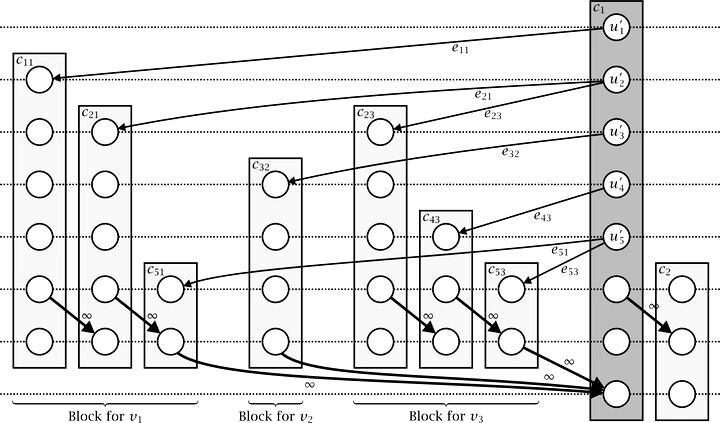# Crossings in Clustered Level Graphs### Abstract

Clustered graphs are an enhanced graph model with a recursive clustering of the vertices according to a given nesting relation. This prime technique for expressing coherence of certain parts of the graph is used in many applications, such as biochemical pathways and UML class diagrams. For directed clustered graphs usually level drawings are used, leading to clustered level graphs. In this thesis we analyze the interrelation of clusters and levels and their influence on edge crossings and cluster/edge crossings.

We present a new method for the application of two-level crossing reduction algorithms to clustered level graphs. Our approach is optimal in the sense that it does not introduce unnecessary crossings, and therefore produces fewer crossings as previous results. In contrast to other approaches, our extension scheme retains the optimality of a one-sided two-level crossing reduction algorithm when extended to clustered level graphs.

We also give a new algorithm for constrained one-sided two-level crossing reduction in level graphs, which appears as a subproblem in clustered crossing reduction. Here, the relative position of some vertex pairs on the second level is fixed. Based on the barycenter heuristic, we present a new algorithm that runs in quadratic time and generates fewer crossings than existing simple extensions. It is significantly faster than previous advanced algorithms, while it compares well in terms of crossing number and is easy to implement.

Minimizing crossings also leads to the concept of planarity. Planar drawings are easy to understand and thus preferable to non-planar drawings. Variations of planarity have been studied intensively for level graphs and clustered graphs. We combine these concepts and analyze a new problem: clustered level planarity. We give an efficient algorithm that decides clustered level planarity of elementary clustered level graphs and computes a clustered level embedding, if one exists.

Type
Publication
PhD thesis, University of Passau